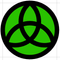cancel
Showing results for
Did you mean:
cancel
Showing results for
Did you mean:
Developer forum
GDL coding questions, Python and add-on development using the API Dev Kit.

## Swap A and B parametersExpert

Tbh I feel silly to ask such basic question, but I can't figure out how to swap values for A, B.

If additional parametes are created it's easy to change their values based on constant A, B. Though how to do it when with help of 2 parameters only? preferably main A, B.

AC 22, 24 | Win 10
13 REPLIES 13If I change the script to the following, it works for me (AC 22 and 26 on macOS)

``````!PARAMETER SCRIPT
!Create values for bSwap (boolean) and A1 & B1 (real) in the parameters

A1 = A
B1 = B

PARAMETERS A1 = A1, B1 = B1

IF GLOB_MODPAR_NAME = "bSwap" THEN

IF bSwap = 1 THEN
A = B1
B = A1
ZZYZX = A1
ELSE
A = A1
B = B1
ZZYZX = B1
ENDIF

PARAMETERS A = A, B = B, ZZYZX = ZZYZX, bSwap = 0

ENDIF``````
Joachim Suehlo . AC12-26 . MAC OSX 10.15 . WIN10Logic failure on my part. 🙄 Without setting bSwap back to Zero the swap would be constantly valid and would try to change A & B every time the Parameter script was run.

So @A_ Smith a working solution...

``````!PARAMETER SCRIPT
!Create values for bSwap (boolean) and A1 & B1 (real) in the parameters

IF GLOB_MODPAR_NAME = "bSwap" THEN

A1 = A
B1 = B
PARAMETERS A1 = A1, B1 = B1

IF bSwap = 1 THEN
A = B1
B = A1
ENDIF

bSwap = 0

PARAMETERS A = A, B = B, bSwap = bSwap

ENDIF``````

OR use @Joachim Suehlo earlier solution which I missed due to this stupid forum formatting.

Apple iMac macOS Ventura / AC26UKI (most recent builds)Indeed. Thanks.

Though, my brain still can't except that this works only due to overriding bSwap - "parameters bSwap=0". Without it should work too, right? just instead I would have to 'unckeck' bSwap and then make it true again (but that doesn't work).

AC 22, 24 | Win 10If you wanted to, you could double the script within the ModPar with IF bSwap = 1 then Else endIF. By doing this it should just keep swapping each time you check it.

 AC22-23 AUS 7000 Help Those Help You - Add a Signature Self-taught, bend it till it breaks Creating a Thread Win10 | R5 2600 | 16GB | GTX1660Latest solutions

Most liked posts